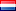##### Approximation of 3D trapezoidal fuzzy data using radial basis functions
Publication Type
Original research
Authors
Fulltext

We present a new methodology to approximate a trapezoidal fuzzy numbers set by using smoothing radial basis functions (RBFs). The methodology uses different error and similarity indices to determine and compare the accuracy of the approximation of the given trapezoidal fuzzy data. For the proposed approximation method a fuzzy radial basis functions type are defined, called fuzzy smoothing radial basis functions under tension. The computation of one of these approximation functions from a given trapezoidal fuzzy data set is described and some convergence results are proved. Finally, some examples in two-dimensions are given to compare the behavior of the presented method by using the proposed error and similarity indices for different configurations of the fuzzy smoothing radial basis functions under tension.

Journal
Title
Fuzzy Sets and Systems
Publisher
Elsiver
Publisher Country
NetherlandsIndexing
Thomson Reuters
Impact Factor
3.343
Publication Type
Both (Printed and Online)
Volume
--
Year
--
Pages
--